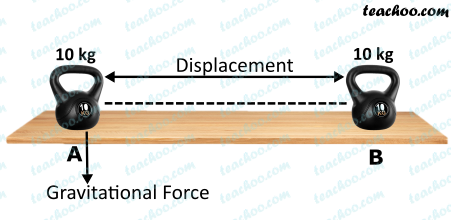NCERT Questions

Class 9
Chapter 11 Class 9 - Work and Energy

## A mass of 10 kg is at a point A on a table. It is moved to a point B. If the line joining A and B is horizontal, what is the work done on the object by the gravitational force? Explain your answer.

###googletag.cmd.push(function() { googletag.display('div-gpt-ad-1669298377854-0'); });

Work is done when force applied produces motion in the direction of the force.

In this case,

Force is gravitational force, which is in downward direction

But the displacement of the body is in the horizontal direction.

Since angle between force and displacement is 90 degrees.

Hence, no work is done

Learn in your speed, with individual attention - Teachoo Maths 1-on-1 Class